Finding sum of n terms

Chapter 5 Class 10 Arithmetic Progressions (Term 2)
Concept wise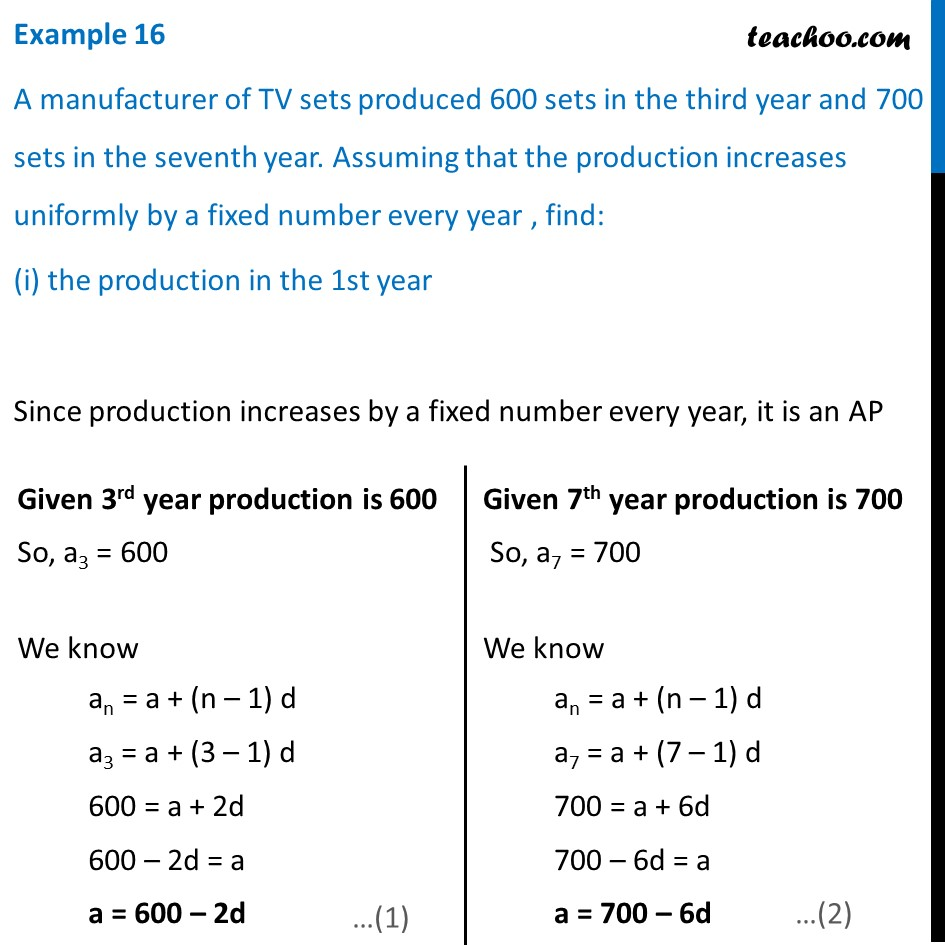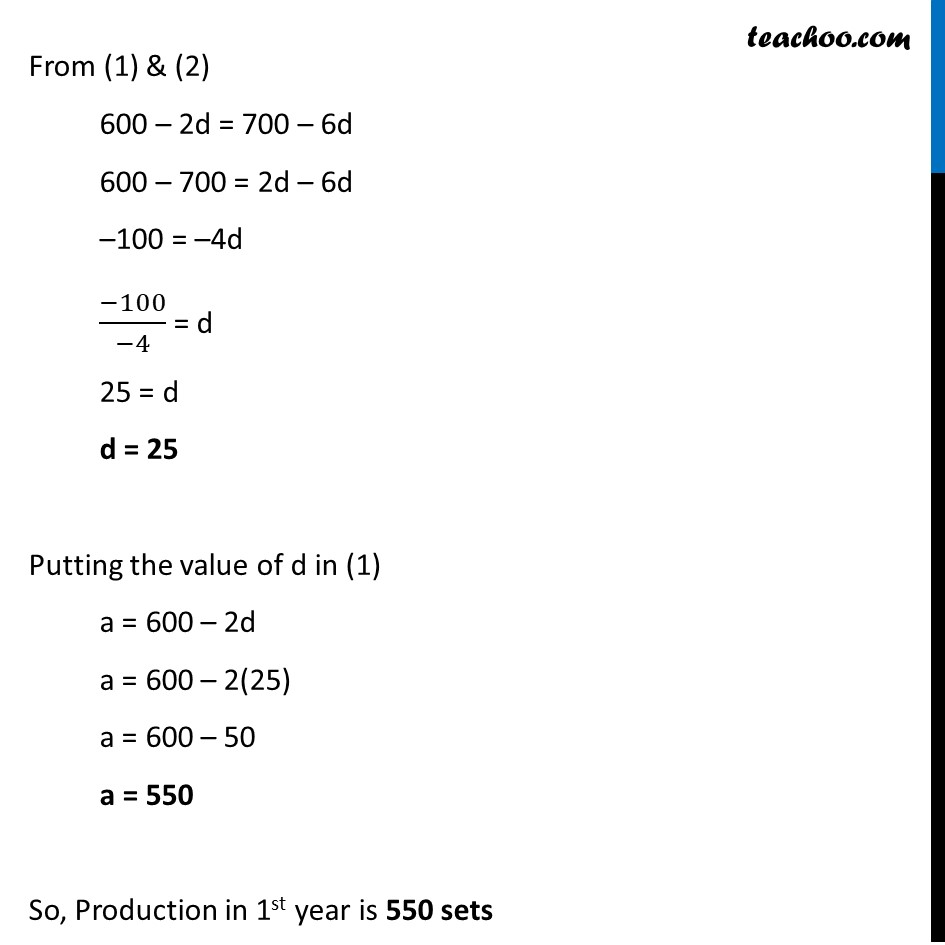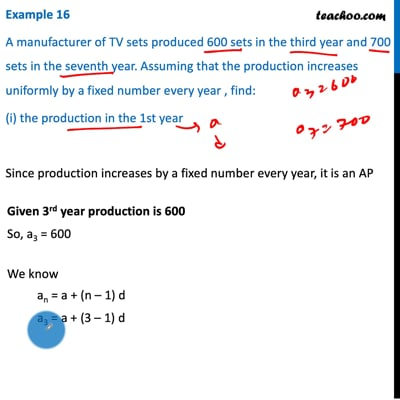This video is only available for Teachoo black users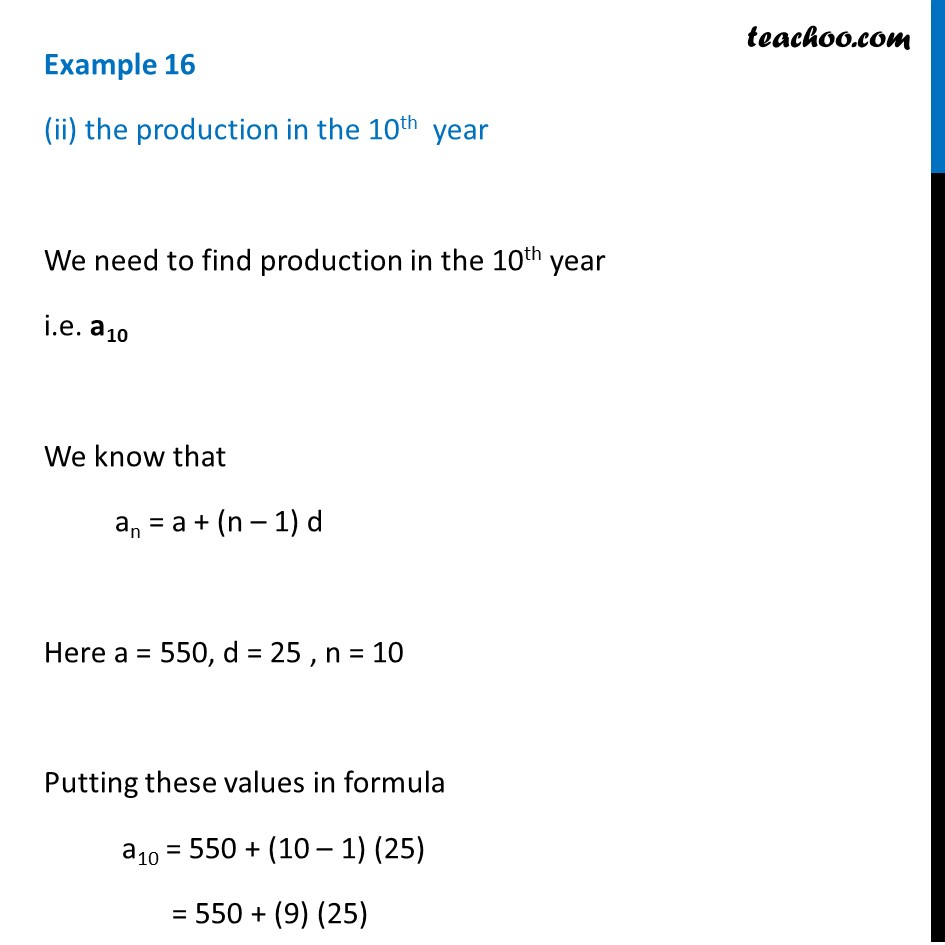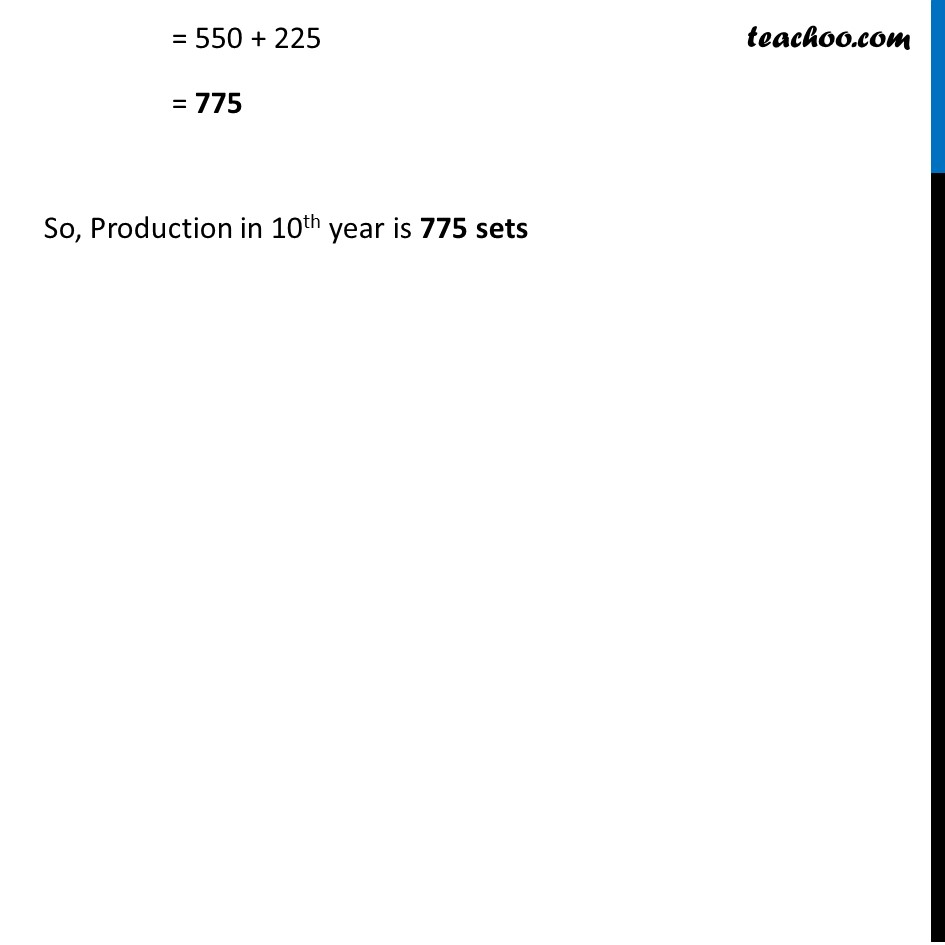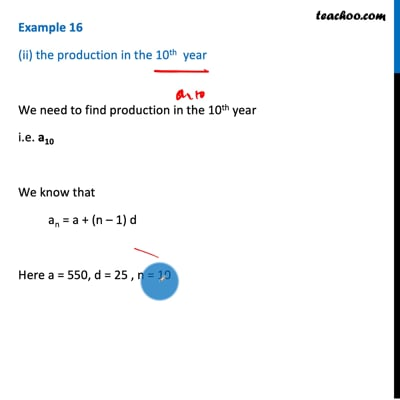This video is only available for Teachoo black users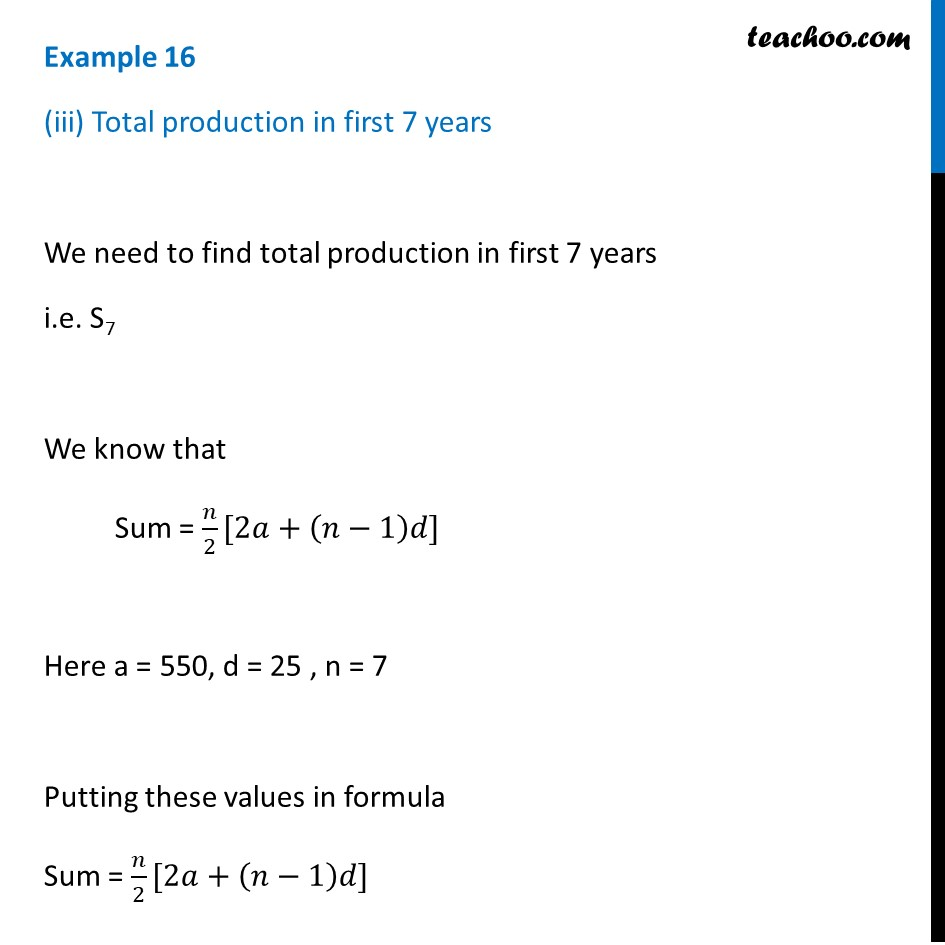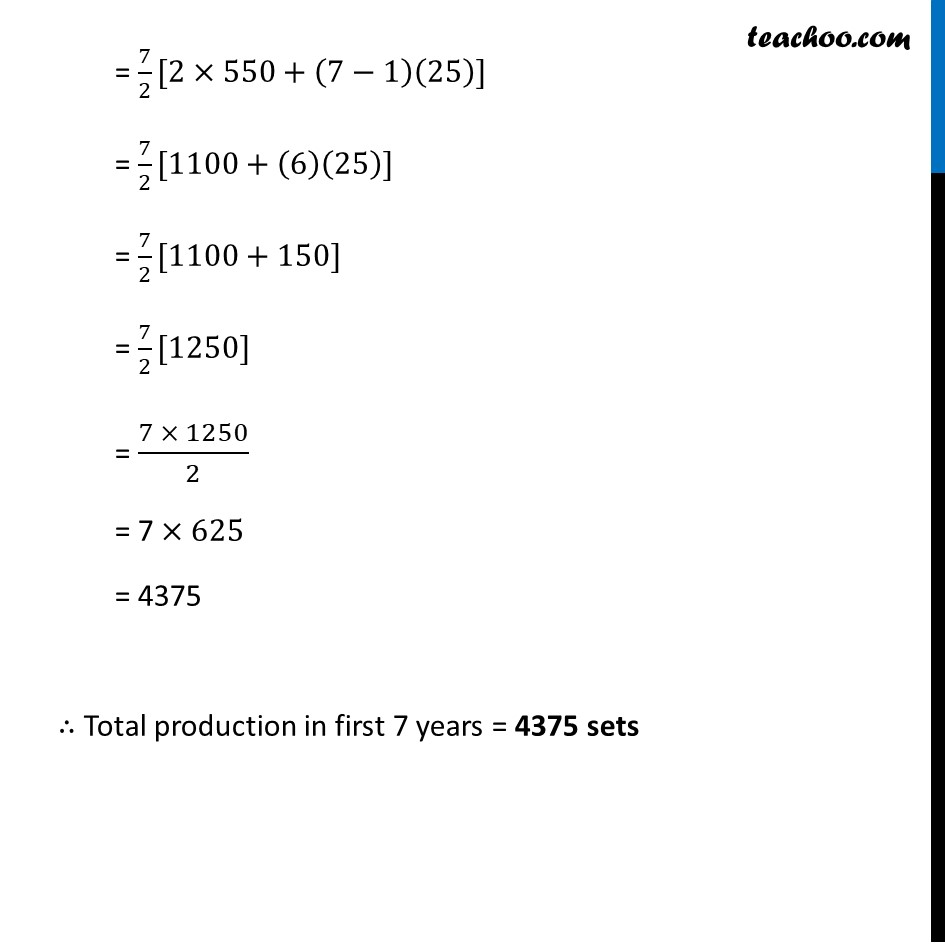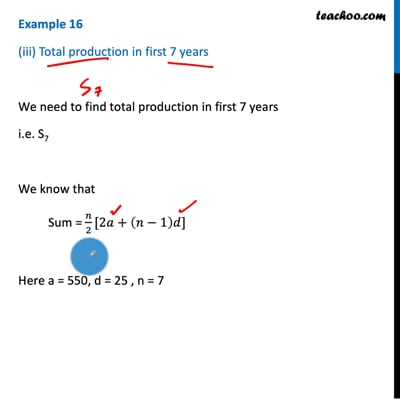This video is only available for Teachoo black users

### Transcript

Example 16 A manufacturer of TV sets produced 600 sets in the third year and 700 sets in the seventh year. Assuming that the production increases uniformly by a fixed number every year , find: (i) the production in the 1st year Since production increases by a fixed number every year, it is an AP Given 3rd year production is 600 So, a3 = 600 We know an = a + (n – 1) d a3 = a + (3 – 1) d 600 = a + 2d 600 – 2d = a a = 600 – 2d Given 7th year production is 700 So, a7 = 700 We know an = a + (n – 1) d a7 = a + (7 – 1) d 700 = a + 6d 700 – 6d = a a = 700 – 6d From (1) & (2) 600 – 2d = 700 – 6d 600 – 700 = 2d – 6d –100 = –4d (−100)/(−4) = d 25 = d d = 25 Putting the value of d in (1) a = 600 – 2d a = 600 – 2(25) a = 600 – 50 a = 550 So, Production in 1st year is 550 sets Example 16 (ii) the production in the 10th year We need to find production in the 10th year i.e. a10 We know that an = a + (n – 1) d Here a = 550, d = 25 , n = 10 Putting these values in formula a10 = 550 + (10 – 1) (25) = 550 + (9) (25) = 550 + 225 = 775 So, Production in 10th year is 775 sets Example 16 (iii) Total production in first 7 years We need to find total production in first 7 years i.e. S7 We know that Sum = 𝑛/2[2𝑎+(𝑛−1)𝑑] Here a = 550, d = 25 , n = 7 Putting these values in formula Sum = 𝑛/2[2𝑎+(𝑛−1)𝑑] = 7/2[2×550+(7−1)(25)] = 7/2[1100+(6)(25)] = 7/2[1100+150] = 7/2 = (7 × 1250)/2 = 7 ×625 = 4375 ∴ Total production in first 7 years = 4375 sets